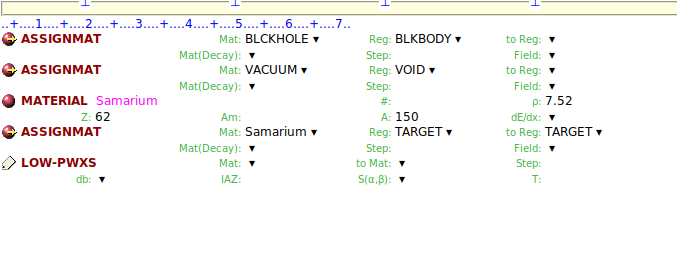# Query regarding different options in USRYIELD

Dear expert,

In the present simulation, a Sm 150 target is hit by 28 MeV alpha. The intention is to get distribution of d2 sigma / dE d (omega). In this regard, I have the following queries:

1. There are two d2 sigma / dx1 dx2 in the Kind option. If I choose the first one, then in Mat card, nothing is getting selected. But if I choose the second one, then in Mat, I can choose Samarium. Can you please explain the difference between two options?

2. The neutrons are produced from the compound nucleus of Gadolinium . So, do I have to choose Gd as material in USRYIELD?

3. Here in yields, there are two variables. For the second variable, although we can give min and max range, but there is no bin number for the second variable. Since I have used polar angle, will it still represent omega i.e. solid angle? If yes, then this d(omega) is just the value Max2 - Min 2, right ?

4. I have added Samarium material separately with A = 150. Earlier, when we used LOW-MAT card, there were only few options for the associated material , for e.g. natural Sm for two temperatures. Now, since we are using a blank LOW-PWXS, will it automatically choose A = 150 ? Since my intention is to get neutron spectrum for A = 154 also later on.Regards,
Riya

1. Actually the first one should be removed from the Flair list (it’s a bug).
2. Not at all. In your case, the choice of the material in USRYIELD is meant to multiply the reaction product yield (d2N/dx1dx2) by the reaction cross section of the beam particle in the selected material. So it should be Samarium (monoisotopic A=150 as in your MATERIAL card).
3. No. Max2 and Min2 are polar angle values, according to your own choice. The result is divided by the respective dOmega [solid angle], which is not (Max2-Min2) [polar angle]: for a polar angle interval from 0 (Min2) to pi (Max2), dOmega is 4pi.
4. Yes.

Thank you @ceruttif for the clarification.

To make my concept clear, the reaction cross section of the beam particle (with a particular energy E ) in the selected material is not a fixed value, right ? For e.g., the production yields of neutrons are different at different energies due to fact that their origin lies in different energy levels (excitation energy) of residual/ compound nucleus. Hence accordingly for each case (i.e. residuals with different excitation energy) the corresponding reaction cross section will be considered while estimating d2 (sigma). Is it so ?

Regards,
Riya

The reaction cross section of the beam particle at the beam energy in a specific material is a fixed value, which is the one used for the USRYIELD result normalization. Hence the use of the d2sigma option of USRYIELD is appropriate only when your setup is such as reactions take place at the beam energy, and not at lower energies (due to beam energy loss) or induced by other particles. In the latter case (thick targets), it makes no sense and you cannot calculate cross sections.
For getting cross sections at different energies, you have to change the beam energy and run different cases.
Also, note that the reaction cross section is related to the probability that a reaction takes place, not a specific product is generated. The production cross section is given by the multiplication of the specific product yield per reaction by the reaction cross section, which is what the considered USRYIELD option does.
In general, FLUKA is not designed to extract microscopic cross sections, although under certain circumstances they can be obtained.

1 Like

Thank you @ceruttif , the topic is clear to me.

Dear @ceruttif ,

If I understood correctly, the angle in usryield is defined with respect to incident beam. So in the attached image, if a detector is placed at C location, then the corresponding usryield angle wil be angle BOC, instead of angle AOC. Is it correct ?

Is there any way to verify/ visualise this region in output ?

Regards,
Riya

Correct.

I do not understand what you mean.
You have the geometry visualization in Flair (thanks to the geoviewer module).
And the USRYIELD scoring parameters are echoed in output.

Thank you @ceruttif for the clarification.

Regards,
Riya